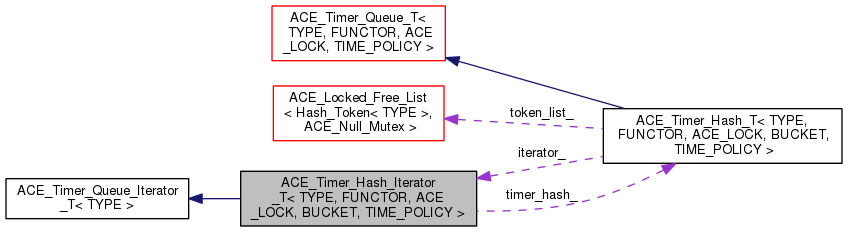ACE  6.1.2
ACE_Timer_Hash_Iterator_T< TYPE, FUNCTOR, ACE_LOCK, BUCKET, TIME_POLICY > Class Template Reference

Iterates over an ACE_Timer_Hash_T. More...

`#include <Timer_Hash_T.h>`

Inheritance diagram for ACE_Timer_Hash_Iterator_T< TYPE, FUNCTOR, ACE_LOCK, BUCKET, TIME_POLICY >:[legend]
Collaboration diagram for ACE_Timer_Hash_Iterator_T< TYPE, FUNCTOR, ACE_LOCK, BUCKET, TIME_POLICY >:[legend]

List of all members.

## Public Types

typedef ACE_Timer_Hash_T< TYPE,
FUNCTOR, ACE_LOCK, BUCKET,
TIME_POLICY >
Hash
Constructor.

## Public Member Functions

ACE_Timer_Hash_Iterator_T (Hash &)
virtual ~ACE_Timer_Hash_Iterator_T ()
virtual void first (void)
Positions the iterator at the earliest node in the Timer Queue.
virtual void next (void)
Positions the iterator at the next node in the Timer Queue.
virtual bool isdone (void) const
Returns true when there are no more nodes in the sequence.
virtual ACE_Timer_Node_T< TYPE > * item (void)
Returns the node at the current position in the sequence.

## Protected Attributes

Hashtimer_hash_
Pointer to the ACE_Timer_Hash that we are iterating over.
size_t position_
Current position in <timer_hash_>'s table.
ACE_Timer_Queue_Iterator_T
< TYPE > *
iter_
Current iterator used on <position>'s bucket.

## Detailed Description

### template<class TYPE, class FUNCTOR, class ACE_LOCK, class BUCKET, typename TIME_POLICY = ACE_Default_Time_Policy> class ACE_Timer_Hash_Iterator_T< TYPE, FUNCTOR, ACE_LOCK, BUCKET, TIME_POLICY >

Iterates over an ACE_Timer_Hash_T.

This is a generic iterator that can be used to visit every node of a timer queue. Be aware that it doesn't transverse in the order of timeout values.

## Member Typedef Documentation

template<class TYPE , class FUNCTOR , class ACE_LOCK , class BUCKET , typename TIME_POLICY = ACE_Default_Time_Policy>
 typedef ACE_Timer_Hash_T ACE_Timer_Hash_Iterator_T< TYPE, FUNCTOR, ACE_LOCK, BUCKET, TIME_POLICY >::Hash

Constructor.

## Constructor & Destructor Documentation

template<class TYPE , class FUNCTOR , class ACE_LOCK , class BUCKET , typename TIME_POLICY >
 ACE_Timer_Hash_Iterator_T< TYPE, FUNCTOR, ACE_LOCK, BUCKET, TIME_POLICY >::ACE_Timer_Hash_Iterator_T ( Hash & hash )
template<class TYPE , class FUNCTOR , class ACE_LOCK , class BUCKET , typename TIME_POLICY >
 ACE_Timer_Hash_Iterator_T< TYPE, FUNCTOR, ACE_LOCK, BUCKET, TIME_POLICY >::~ACE_Timer_Hash_Iterator_T ( )
virtual

## Member Function Documentation

template<class TYPE , class FUNCTOR , class ACE_LOCK , class BUCKET , typename TIME_POLICY >
 void ACE_Timer_Hash_Iterator_T< TYPE, FUNCTOR, ACE_LOCK, BUCKET, TIME_POLICY >::first ( void )
virtual

Positions the iterator at the earliest node in the Timer Queue.

Implements ACE_Timer_Queue_Iterator_T< TYPE >.

template<class TYPE , class FUNCTOR , class ACE_LOCK , class BUCKET , typename TIME_POLICY >
 bool ACE_Timer_Hash_Iterator_T< TYPE, FUNCTOR, ACE_LOCK, BUCKET, TIME_POLICY >::isdone ( void ) const
virtual

Returns true when there are no more nodes in the sequence.

Implements ACE_Timer_Queue_Iterator_T< TYPE >.

template<class TYPE , class FUNCTOR , class ACE_LOCK , class BUCKET , typename TIME_POLICY >
 ACE_Timer_Node_T< TYPE > * ACE_Timer_Hash_Iterator_T< TYPE, FUNCTOR, ACE_LOCK, BUCKET, TIME_POLICY >::item ( void )
virtual

Returns the node at the current position in the sequence.

Implements ACE_Timer_Queue_Iterator_T< TYPE >.

template<class TYPE , class FUNCTOR , class ACE_LOCK , class BUCKET , typename TIME_POLICY >
 void ACE_Timer_Hash_Iterator_T< TYPE, FUNCTOR, ACE_LOCK, BUCKET, TIME_POLICY >::next ( void )
virtual

Positions the iterator at the next node in the Timer Queue.

Implements ACE_Timer_Queue_Iterator_T< TYPE >.

## Member Data Documentation

template<class TYPE , class FUNCTOR , class ACE_LOCK , class BUCKET , typename TIME_POLICY = ACE_Default_Time_Policy>
 ACE_Timer_Queue_Iterator_T* ACE_Timer_Hash_Iterator_T< TYPE, FUNCTOR, ACE_LOCK, BUCKET, TIME_POLICY >::iter_
protected

Current iterator used on <position>'s bucket.

template<class TYPE , class FUNCTOR , class ACE_LOCK , class BUCKET , typename TIME_POLICY = ACE_Default_Time_Policy>
 size_t ACE_Timer_Hash_Iterator_T< TYPE, FUNCTOR, ACE_LOCK, BUCKET, TIME_POLICY >::position_
protected

Current position in <timer_hash_>'s table.

template<class TYPE , class FUNCTOR , class ACE_LOCK , class BUCKET , typename TIME_POLICY = ACE_Default_Time_Policy>
 Hash& ACE_Timer_Hash_Iterator_T< TYPE, FUNCTOR, ACE_LOCK, BUCKET, TIME_POLICY >::timer_hash_
protected

Pointer to the ACE_Timer_Hash that we are iterating over.

The documentation for this class was generated from the following files: